# sklearn.cluster.ward_tree¶

sklearn.cluster.ward_tree(X, connectivity=None, n_components=None, n_clusters=None, return_distance=False)[源代码]

Ward clustering based on a Feature matrix.

Recursively merges the pair of clusters that minimally increases within-cluster variance.

The inertia matrix uses a Heapq-based representation.

This is the structured version, that takes into account some topological structure between samples.

Read more in the User Guide.

Parameters: X : array, shape (n_samples, n_features) feature matrix representing n_samples samples to be clustered connectivity : sparse matrix (optional). connectivity matrix. Defines for each sample the neighboring samples following a given structure of the data. The matrix is assumed to be symmetric and only the upper triangular half is used. Default is None, i.e, the Ward algorithm is unstructured. n_components : int (optional) Number of connected components. If None the number of connected components is estimated from the connectivity matrix. NOTE: This parameter is now directly determined directly from the connectivity matrix and will be removed in 0.18 n_clusters : int (optional) Stop early the construction of the tree at n_clusters. This is useful to decrease computation time if the number of clusters is not small compared to the number of samples. In this case, the complete tree is not computed, thus the ‘children’ output is of limited use, and the ‘parents’ output should rather be used. This option is valid only when specifying a connectivity matrix. return_distance: bool (optional) : If True, return the distance between the clusters. children : 2D array, shape (n_nodes-1, 2) The children of each non-leaf node. Values less than n_samples correspond to leaves of the tree which are the original samples. A node i greater than or equal to n_samples is a non-leaf node and has children children_[i - n_samples]. Alternatively at the i-th iteration, children[i] and children[i] are merged to form node n_samples + i n_components : int The number of connected components in the graph. n_leaves : int The number of leaves in the tree parents : 1D array, shape (n_nodes, ) or None The parent of each node. Only returned when a connectivity matrix is specified, elsewhere ‘None’ is returned. distances : 1D array, shape (n_nodes-1, ) Only returned if return_distance is set to True (for compatibility). The distances between the centers of the nodes. distances[i] corresponds to a weighted euclidean distance between the nodes children[i, 1] and children[i, 2]. If the nodes refer to leaves of the tree, then distances[i] is their unweighted euclidean distance. Distances are updated in the following way (from scipy.hierarchy.linkage): The new entry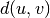is computed as follows,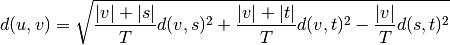where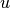is the newly joined cluster consisting of clusters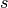and,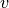is an unused cluster in the forest,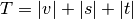, and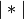is the cardinality of its argument. This is also known as the incremental algorithm.